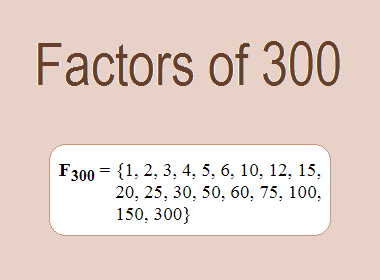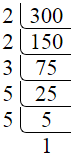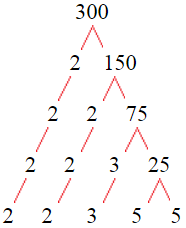# Factors of 300The factors of 300 are 1, 2, 3, 4, 5, 6, 10, 12, 15, 20, 25, 30, 50, 60, 75, 100, 150, and 300 i.e. F300 = {1, 2, 3, 4, 5, 6, 10, 12, 15, 20, 25, 30, 50, 60, 75, 100, 150, 300}. The factors of 300 are all the numbers that can divide 300 without leaving a remainder.

We can check if these numbers are factors of 300 by dividing 300 by each of them. If the result is a whole number, then the number is a factor of 300. Let's do this for each of the numbers listed above:

·        1 is a factor of 300 because 300 divided by 1 is 300.

·        2 is a factor of 300 because 300 divided by 2 is 150.

·        3 is a factor of 300 because 300 divided by 3 is 100.

·        4 is a factor of 300 because 300 divided by 4 is 75.

·        5 is a factor of 300 because 300 divided by 5 is 60.

·        6 is a factor of 300 because 300 divided by 6 is 50.

·        10 is a factor of 300 because 300 divided by 10 is 30.

·        12 is a factor of 300 because 300 divided by 12 is 25.

·        15 is a factor of 300 because 300 divided by 15 is 20.

·        20 is a factor of 300 because 300 divided by 20 is 15.

·        25 is a factor of 300 because 300 divided by 25 is 12.

·        30 is a factor of 300 because 300 divided by 30 is 10.

·        50 is a factor of 300 because 300 divided by 50 is 6.

·        60 is a factor of 300 because 300 divided by 60 is 5.

·        75 is a factor of 300 because 300 divided by 75 is 4.

·        100 is a factor of 300 because 300 divided by 100 is 3.

·        150 is a factor of 300 because 300 divided by 150 is 2.

·        300 is a factor of 300 because 300 divided by 300 is 1.

## How to Find Factors of 300?

1 and the number itself are the factors of every number. So, 1 and 300 are two factors of 300. To find the other factors of 300, we can start by dividing 300 by the numbers between 1 and 300. If we divide 300 by 2, we get a remainder of 0. Therefore, 2 is a factor of 300. If we divide 300 by 3, we get a remainder of 0. Therefore, 3 is also a factor of 300.

Next, we can check if 4 is a factor of 300. If we divide 300 by 4, we get a remainder of 0. Therefore, 4 is also a factor of 300. We can continue this process for all the possible factors of 300.

Through this process, we can find that the factors of 300 are 1, 2, 3, 4, 5, 6, 10, 12, 15, 20, 25, 30, 50, 60, 75, 100, 150, and 300. These are the only numbers that can divide 300 without leaving a remainder.

********************

********************

## Properties of the Factors of 300

The factors of 300 have some interesting properties. One of the properties is that the sum of the factors of 300 is equal to 868. We can see this by adding all the factors of 300 together:

1 + 2 + 3 + 4 + 5 + 6 + 10 + 12 + 15 + 20 + 25 + 30 + 50 + 60 + 75 + 100 + 150 + 300 = 868

Another property of the factors of 300 is that the prime factors of 300 are 2, 3, and 5 only.

## Applications of the Factors of 300

The factors of 300 have several applications in mathematics. One of the applications is in finding the highest common factor (HCF) of two or more numbers. The HCF is the largest factor that two or more numbers have in common. For example, to find the HCF of 300 and 84, we need to find the factors of both numbers and identify the largest factor they have in common. The factors of 300 are 1, 2, 3, 4, 5, 6, 10, 12, 15, 20, 25, 30, 50, 60, 75, 100, 150, and 300. The factors of 84 are 1, 2, 3, 4, 6, 7, 12, 14, 21, 28, 42, and 84. The largest factor that they have in common is 12. Therefore, the HCF of 300 and 84 is 12.

Another application of the factors of 300 is in prime factorization. Prime factorization is the process of expressing a number as the product of its prime factors. The prime factors of 300 are 2, 3, and 5 since these are the only prime numbers that can divide 300 without leaving a remainder. Therefore, we can express 300 as:

300 = 2 × 2 × 3 × 5 × 5

We can do prime factorization by division and factor tree method also. Here is the prime factorization of 300 by division method,300 = 2 × 2 × 3 × 5 × 5

Here is the prime factorization of 300 by the factor tree method,300 = 2 × 2 × 3 × 5 × 5

## Conclusion

The factors of 300 are the numbers that can divide 300 without leaving a remainder. The factors of 300 are 1, 2, 3, 4, 5, 6, 10, 12, 15, 20, 25, 30, 50, 60, 75, 100, 150, and 300. The factors of 300 have some interesting properties, such as having a sum of 868. The factors of 300 have several applications in mathematics, such as finding the highest common factor and prime factorization.